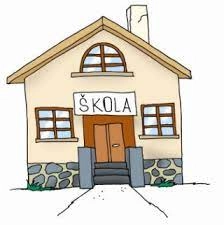# Two classes

The average of 1A class of 25 is 12 years and the average age of 1B class of 22 is 10 years. What is the average age of the pupils of 1A and 1B classes?

x =  11.0638

### Step-by-step explanation:Did you find an error or inaccuracy? Feel free to write us. Thank you!

Tips for related online calculators
Looking for help with calculating arithmetic mean?
Looking for a statistical calculator?

#### Grade of the word problem:

We encourage you to watch this tutorial video on this math problem: